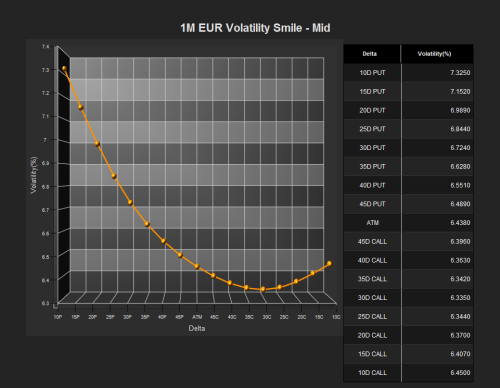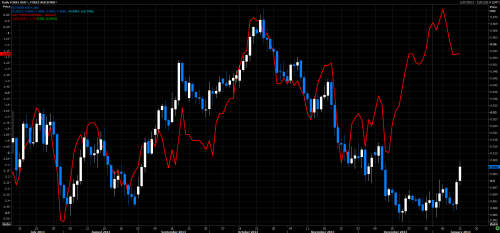Introduction:

Implied volatility(IV or vol) in essence is the expected change in price over a given period and is a useful, if not, slightly peculiar indicator. As IV is a factor in option pricing models with all other things being equal (as in strike price, duration etc) the higher the IV the higher the "price" of the option.

To demonstrate this point clearly we can see below a 1 month EURUSD at the money option contract. On the left hand scale we can see the Implied volatility as a number, often expressed in %. And on the Right scale, we have the premium on the same option.Here, we can seethe premium (price) is 100 pips or 0.01 (in EURUSD quotes) and it can be seen how the premium fluctuates perfectly with Implied Volatility.

So now that we have the basic idea that the IV is correlated to the "price" of the premium we can use this to analyse in various ways.

Risk Reversals:

An FX risk reversal(RRs) is simply put as the difference between the implied volatility between a Put contract and a call contract that are below and above the current spot price respectively. Simply put IV of call - IV of put.

The market standardfor Risk reversals is using the 25 delta contracts. Now this is mostly arbitrary for the analysis, but for ease of understanding I've attached a chart showing the strike prices for 25 delta calls and 25 delta puts.What is crucial from here is the idea the the Put contracts are below spot price and Calls are above. It is also to note for the next image that the lower the delta, the further away from spot the contract strike price is. I.e. 10 delta is farther from Spot than 25 delta and 40 delta etc.

so we bring this onwards and we can construct what is called a Volatility smile for the EURUSD for the 1 month maturity.So here we have IVon the y-axis and the 1 month contracts defined by delta. What we can clearly see is that the smile isn't symmetrical and this leads to how we can analyse the options markets.

Linking back to the idea that IV is proportional to the price of a contract, then we can see that the price of Put contracts is much greater than that of calls. So what does this tell us?

Well simply, there is greater demand for puts than calls - simply because greater demand leads to higher prices. When someone buys a put, that is a bearish trade, and when someone buys a call it is bullish, so this tells us there is more demand to be bearish the EURUSD over the next 1 month than bullish.

BUT, this is a snapshot against a single time period, it is not much use when comparing over time, because you don't know if this line was steeper or shallower than the previous day or week etc etc.So here we have in red the rolling 1 month 25 delta risk reversal (the difference in IV between the 25 delta call and put).

As we can see thereis a very strong correlation, and so there should be. As the line moves up, the market is becoming relatively more bullish, as the change demand for calls is greater than change in demand for puts. This idea can also be easily considered
on the downside too.

Here is another,potentially tradable example for the AUDUSD and AUD 1 month 25D RR (red)So what we can see here is that they were highly correlated until recently. Where the 25D RR all of a sudden stopped following the AUDUSD and started moving higher quite fast. What this means is that options traders were getting very bullish the AUDUSD as the demand for calls was rising relative to puts. This will result in one of two things; either the options market will be right, and the AUDUSD will rise. Or, they are wrong and they will have to cover their positions.

In the first case,the options market is looking for a short squeeze in the spot market, where those short are paying each day a small swap to hold short, and the longer it doesn't drop the more they lose, and so eventually they decide to buy back their positions and as such the spot price rises.

In the latter case,the options markets are very bullish and if they are proved wrong by the time their options expire, they will need to delta and gamma hedge so as to reduce exposure and this will force the price lower as well as the Risk reversal.

Either way, I hope that this article has helped in understanding how one can look at the idea of Implied volatility being proportional to price and then using this to analyse the positioning in the marketplace, and then looking for potential trade ideas based on this.

Thanks for readingand I welcome any questions